# Inductive Learning continued Chapter 19 Slides for Ch

• Slides: 47
Download presentationInductive Learning (continued) Chapter 19 Slides for Ch. 19 by J. C. Latombe 1Learning logical descriptions • The process of constructing a decision tree can be seen as searching the hypothesis space H. The goal is to construct an hypothesis H that explains the data in the training set. • The hypothesis H is a logical description of the form: H: H 1 / H 2 / … / Hn Hi: x Q(x) <=> Ci(x) where Q(x) is the goal predicate and Ci(x) are candidate definitions.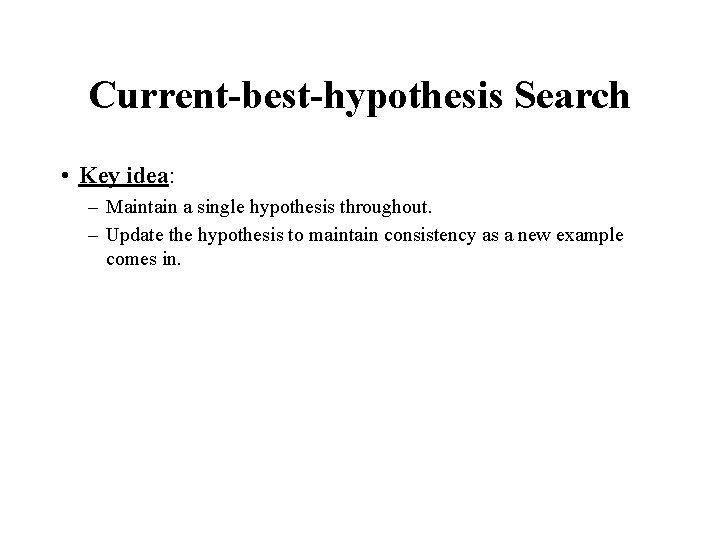Current-best-hypothesis Search • Key idea: – Maintain a single hypothesis throughout. – Update the hypothesis to maintain consistency as a new example comes in.Definitions • Positive example: an instance of the hypothesis • Negative example: not an instance of the hypothesis • False negative example: the hypothesis predicts it should be a negative example but it is in fact positive • False positive example: should be positive but it is actually negative.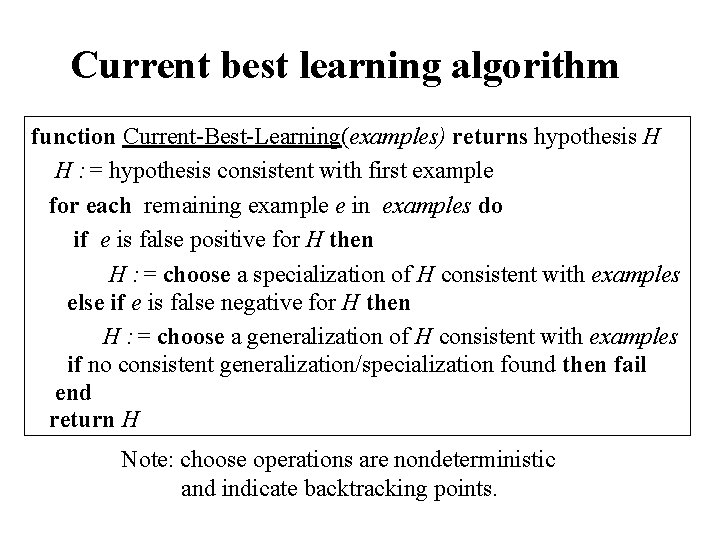Current best learning algorithm function Current-Best-Learning(examples) returns hypothesis H H : = hypothesis consistent with first example for each remaining example e in examples do if e is false positive for H then H : = choose a specialization of H consistent with examples else if e is false negative for H then H : = choose a generalization of H consistent with examples if no consistent generalization/specialization found then fail end return H Note: choose operations are nondeterministic and indicate backtracking points.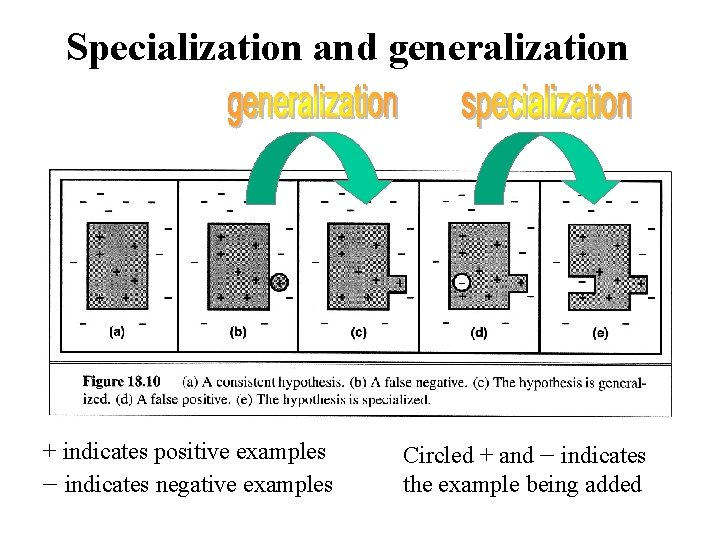Specialization and generalization + indicates positive examples - indicates negative examples Circled + and - indicates the example being addedHow to Generalize a) Replacing Constants with Variables: Object(Animal, Bird) Object (X, Bird) b) Dropping Conjuncts: Object(Animal, Bird) & Feature(Animal, Wings) Object(Animal, Bird) c) Adding Disjuncts: Feature(Animal, Feathers) v Feature(Animal, Fly) d) Generalizing Terms: Feature(Bird, Wings) Feature(Bird, Primary-Feature) http: //www. pitt. edu/~suthers/infsci 1054/8. html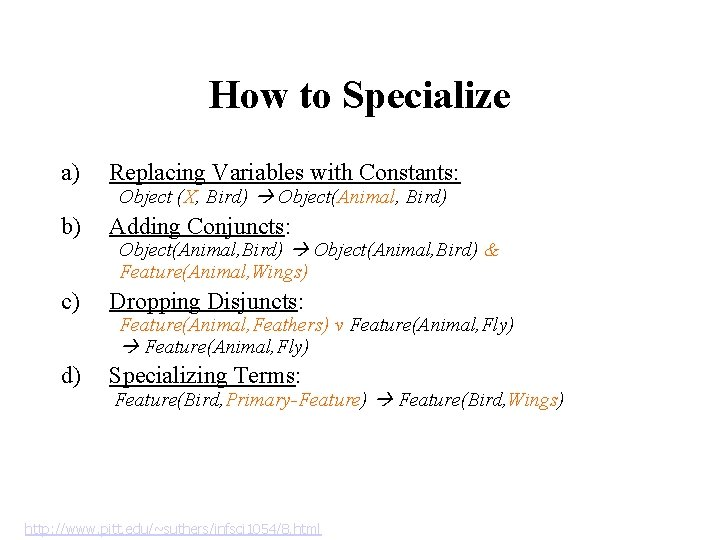How to Specialize a) Replacing Variables with Constants: b) Adding Conjuncts: c) Dropping Disjuncts: d) Specializing Terms: Object (X, Bird) Object(Animal, Bird) & Feature(Animal, Wings) Feature(Animal, Feathers) v Feature(Animal, Fly) Feature(Bird, Primary-Feature) Feature(Bird, Wings) http: //www. pitt. edu/~suthers/infsci 1054/8. htmlDiscussion • The choice of initial hypothesis and specialization and generalization is nondeterministic (use heuristics) • Problems – Extension made not necessarily lead to the simplest hypothesis. – May lead to an unrecoverable situation where no simple modification of the hypothesis is consistent with all of the examples. – What heuristics to use – Could be inefficient (need to check consistency with all examples), and perhaps backtrack or restart – Handling noise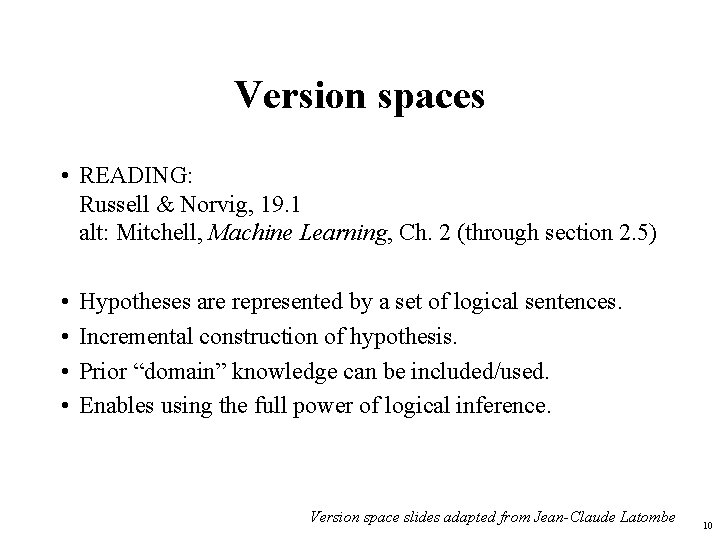Version spaces • READING: Russell & Norvig, 19. 1 alt: Mitchell, Machine Learning, Ch. 2 (through section 2. 5) • • Hypotheses are represented by a set of logical sentences. Incremental construction of hypothesis. Prior “domain” knowledge can be included/used. Enables using the full power of logical inference. Version space slides adapted from Jean-Claude Latombe 10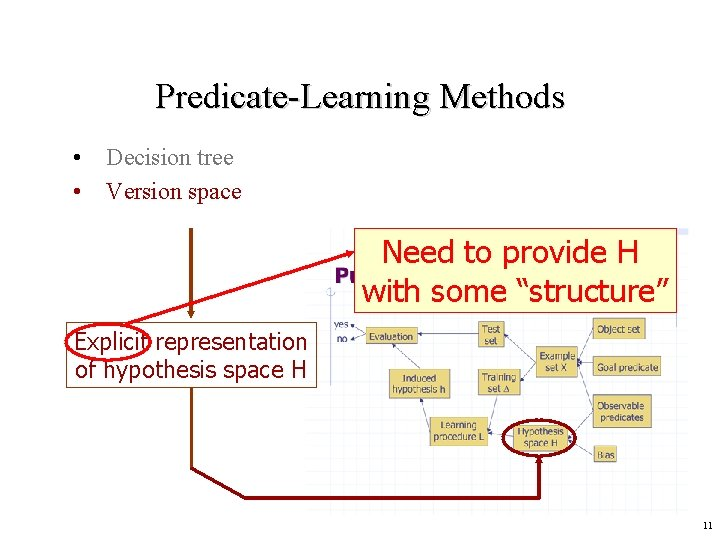Predicate-Learning Methods • Decision tree • Version space Need to provide H with some “structure” Explicit representation of hypothesis space H 11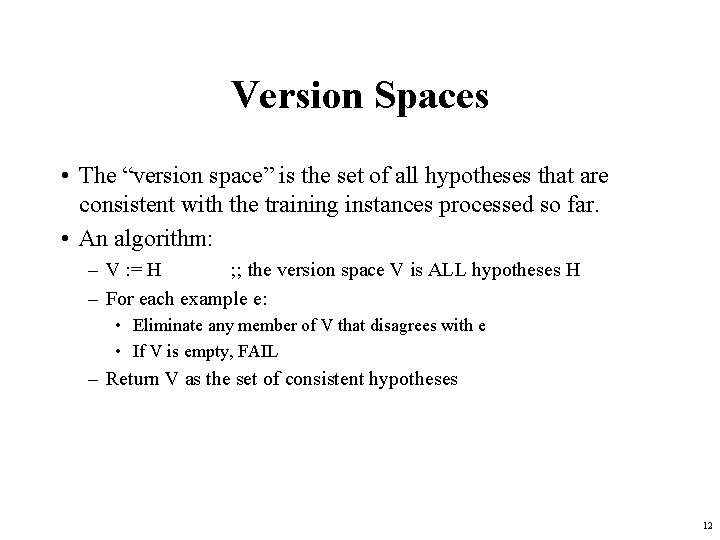Version Spaces • The “version space” is the set of all hypotheses that are consistent with the training instances processed so far. • An algorithm: – V : = H ; ; the version space V is ALL hypotheses H – For each example e: • Eliminate any member of V that disagrees with e • If V is empty, FAIL – Return V as the set of consistent hypotheses 12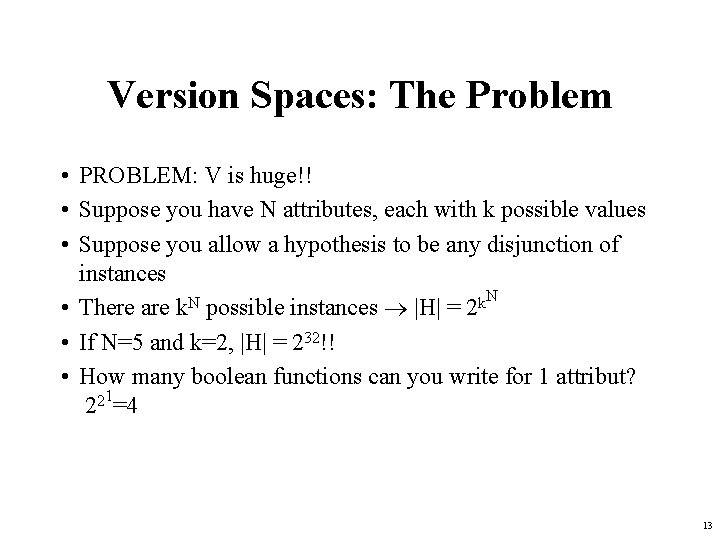Version Spaces: The Problem • PROBLEM: V is huge!! • Suppose you have N attributes, each with k possible values • Suppose you allow a hypothesis to be any disjunction of instances N • There are k. N possible instances |H| = 2 k • If N=5 and k=2, |H| = 232!! • How many boolean functions can you write for 1 attribut? 221=4 13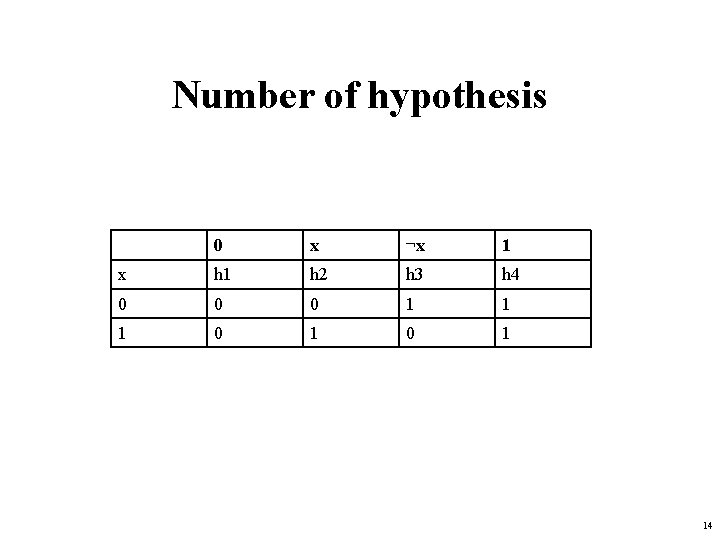Number of hypothesis 0 x ¬x 1 x h 1 h 2 h 3 h 4 0 0 0 1 1 1 0 1 14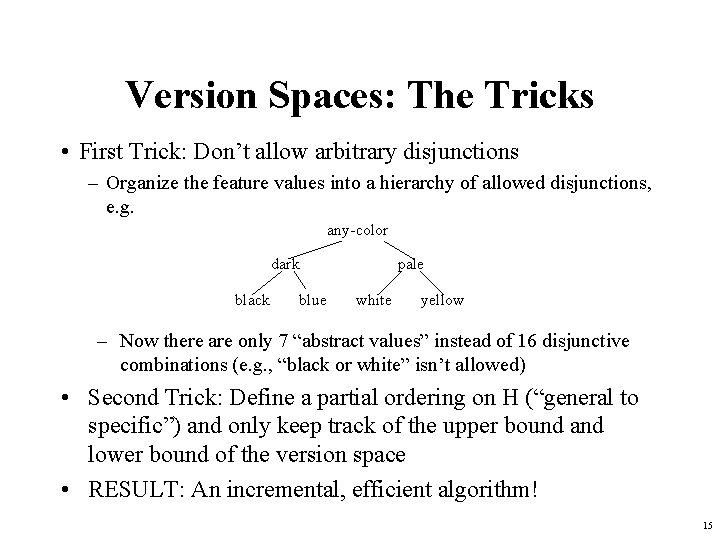Version Spaces: The Tricks • First Trick: Don’t allow arbitrary disjunctions – Organize the feature values into a hierarchy of allowed disjunctions, e. g. any-color dark black blue pale white yellow – Now there are only 7 “abstract values” instead of 16 disjunctive combinations (e. g. , “black or white” isn’t allowed) • Second Trick: Define a partial ordering on H (“general to specific”) and only keep track of the upper bound and lower bound of the version space • RESULT: An incremental, efficient algorithm! 15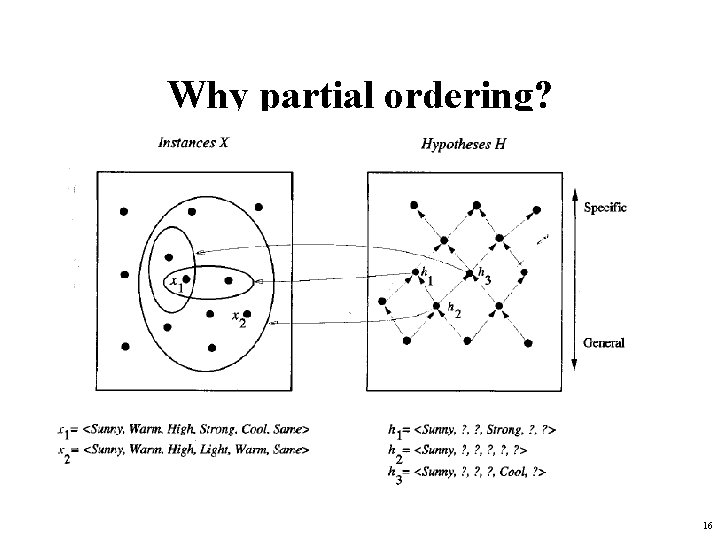Why partial ordering? 16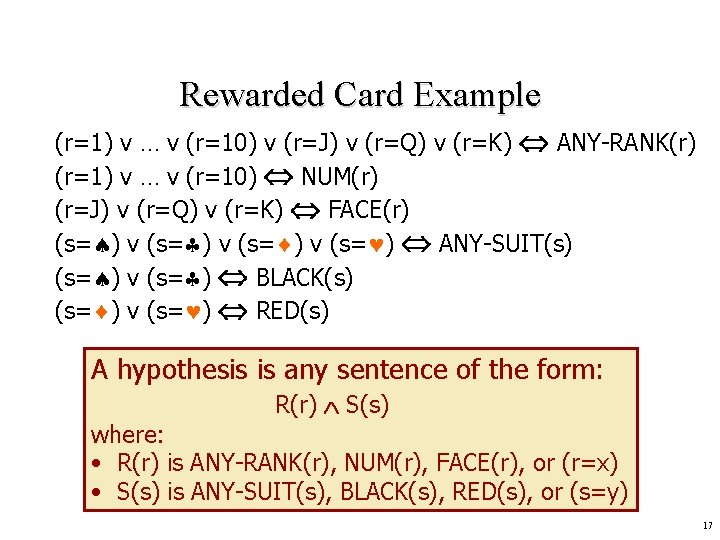Rewarded Card Example (r=1) v … v (r=10) v (r=J) v (r=Q) v (r=K) ANY-RANK(r) (r=1) v … v (r=10) NUM(r) (r=J) v (r=Q) v (r=K) FACE(r) (s= ) v (s= ) ANY-SUIT(s) (s= ) v (s= ) BLACK(s) (s= ) v (s= ) RED(s) A hypothesis is any sentence of the form: R(r) S(s) where: • R(r) is ANY-RANK(r), NUM(r), FACE(r), or (r=x) • S(s) is ANY-SUIT(s), BLACK(s), RED(s), or (s=y) 17Simplified Representation For simplicity, we represent a concept by rs, with: • r {a, n, f, 1, …, 10, j, q, k} • s {a, b, r, , } For example: • n represents: NUM(r) (s= ) • aa represents: ANY-RANK(r) ANY-SUIT(s) 18Extension of a Hypothesis The extension of a hypothesis h is the set of objects that satisfies h Examples: • The extension of f is: {j , q , k } • The extension of aa is the set of all cards 19More General/Specific Relation • Let h 1 and h 2 be two hypotheses in H • h 1 is more general than h 2 iff the extension of h 1 is a proper superset of the extension of h 2 Examples: • aa is more general than f • f is more general than q • fr and nr are not comparable 20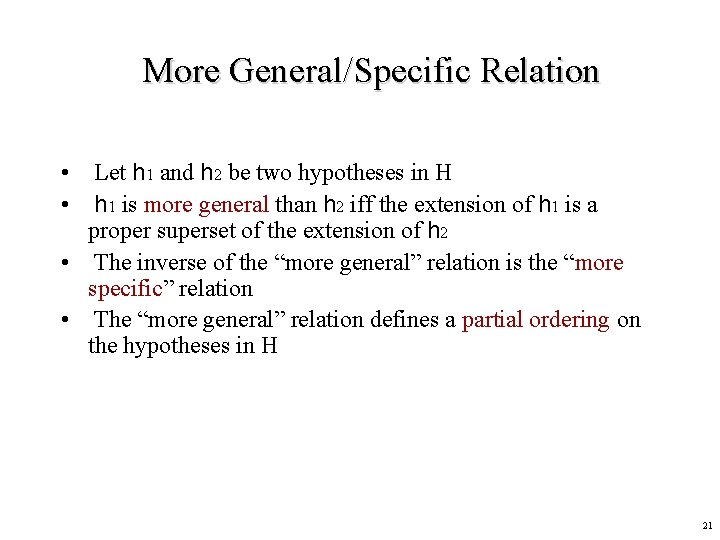More General/Specific Relation • Let h 1 and h 2 be two hypotheses in H • h 1 is more general than h 2 iff the extension of h 1 is a proper superset of the extension of h 2 • The inverse of the “more general” relation is the “more specific” relation • The “more general” relation defines a partial ordering on the hypotheses in H 21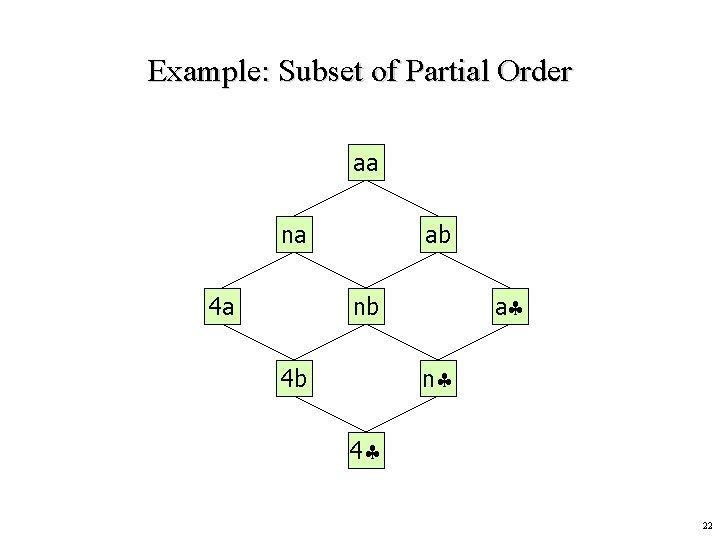Example: Subset of Partial Order aa na 4 a ab a nb n 4 b 4 22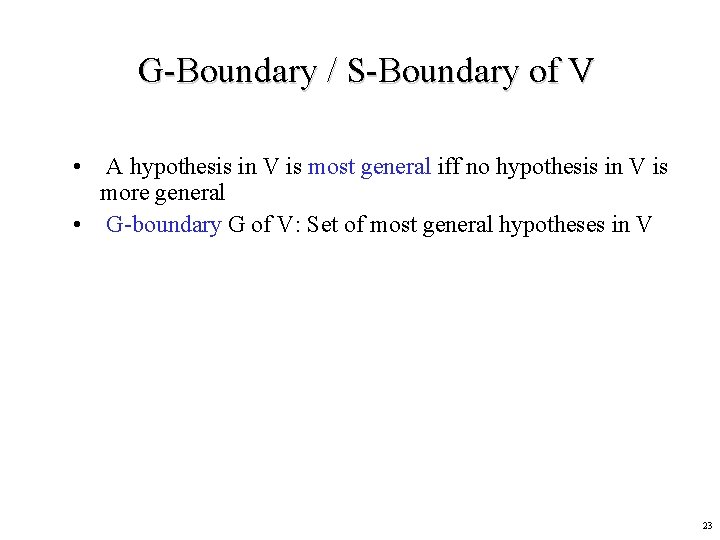G-Boundary / S-Boundary of V • A hypothesis in V is most general iff no hypothesis in V is more general • G-boundary G of V: Set of most general hypotheses in V 23G-Boundary / S-Boundary of V • A hypothesis in V is most general iff no hypothesis in V is more general • G-boundary G of V: Set of most general hypotheses in V • A hypothesis in V is most specific iff no hypothesis in V is more specific • S-boundary S of V: Set of most specific hypotheses in V all inconsistent G 1 G 2 S 1 S 2 G 3 S 3 all inconsistent 24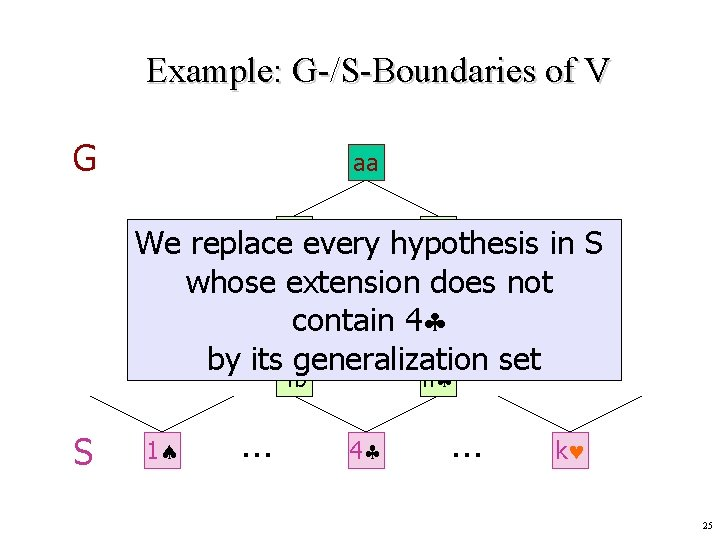Example: G-/S-Boundaries of V G aa ab We replacenaevery hypothesis in S whose extension does Now suppose that 4 not is a 4 a nb 4 example given ascontain a positive by its generalization set n 4 b S 1 … 4 … k 25Example: G-/S-Boundaries of V G aa na G and Sab Here, both have size 1. This is not the case in general! 4 a n 4 b S a nb 4 26Example: G-/S-Boundaries of V na 4 a The aa generalization set of an hypothesis h is the set of the ab hypotheses that are immediately more general than a h nb n 4 b Let 7 be the next (positive) example 4 Generalization set of 4 27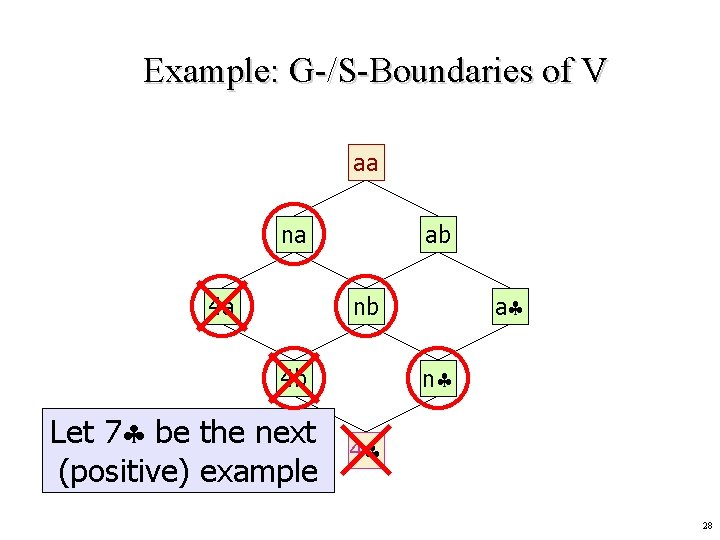Example: G-/S-Boundaries of V aa na 4 a ab a nb n 4 b Let 7 be the next (positive) example 4 28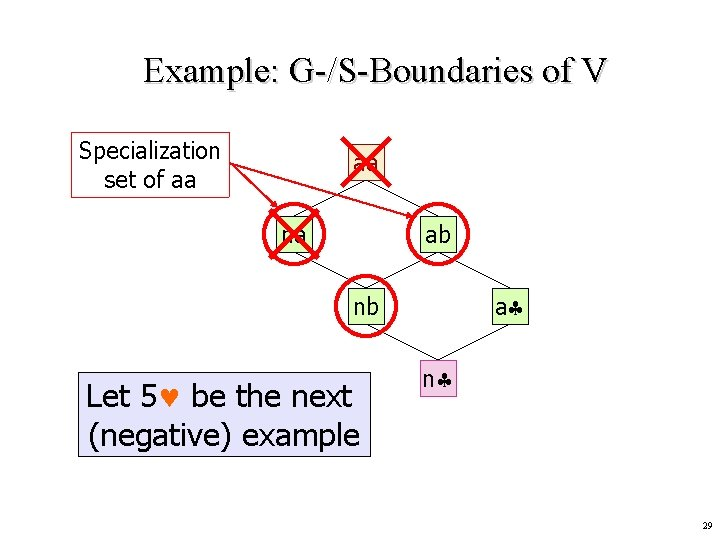Example: G-/S-Boundaries of V Specialization set of aa aa na ab a nb Let 5 be the next (negative) example n 29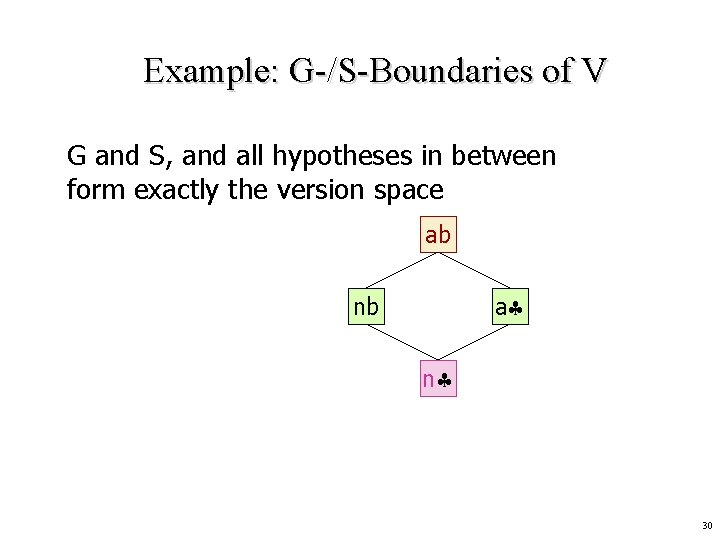Example: G-/S-Boundaries of V G and S, and all hypotheses in between form exactly the version space ab a nb n 30Example: G-/S-Boundaries of V At this stage … ab No Yes Maybe Do 8 , 6 , j satisfy CONCEPT? a nb n 31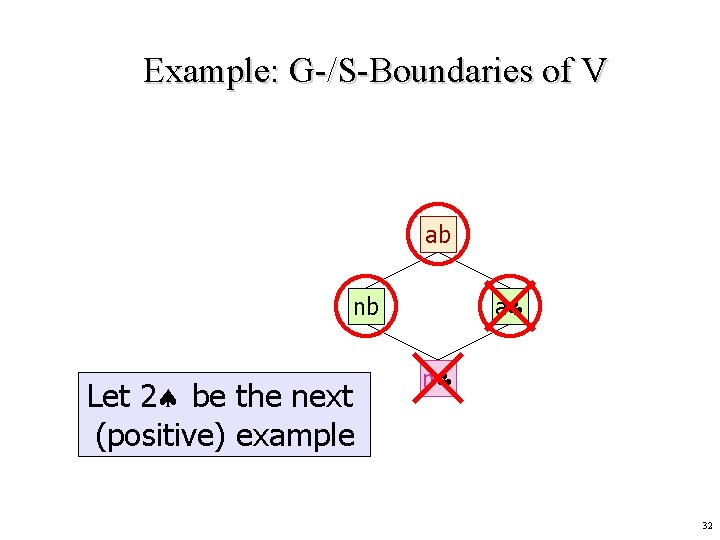Example: G-/S-Boundaries of V ab a nb Let 2 be the next (positive) example n 32Example: G-/S-Boundaries of V ab nb Let j be the next (negative) example 33Example: G-/S-Boundaries of V + 4 7 2 – 5 j nb NUM(r) BLACK(s) 34Example: G-/S-Boundaries of V Let us return to the version space … … and let 8 be the next (negative) example ab nb a The only most specific hypothesis disagrees with n this example, so no hypothesis in H agrees with all examples 35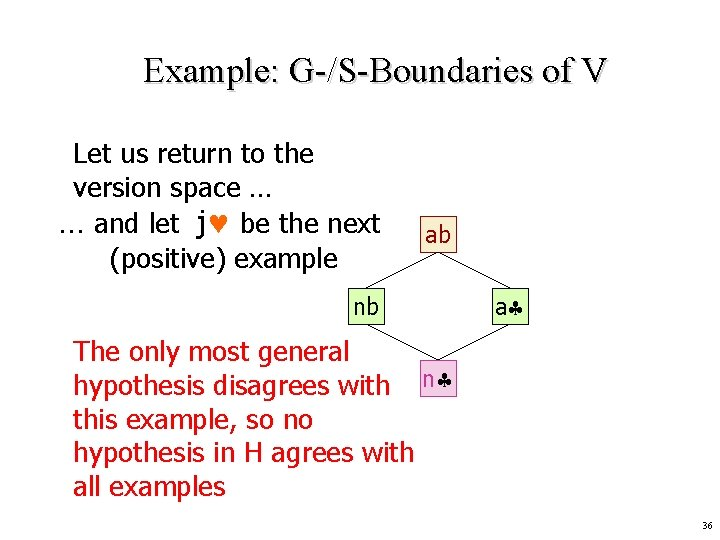Example: G-/S-Boundaries of V Let us return to the version space … … and let j be the next (positive) example ab nb a The only most general hypothesis disagrees with n this example, so no hypothesis in H agrees with all examples 36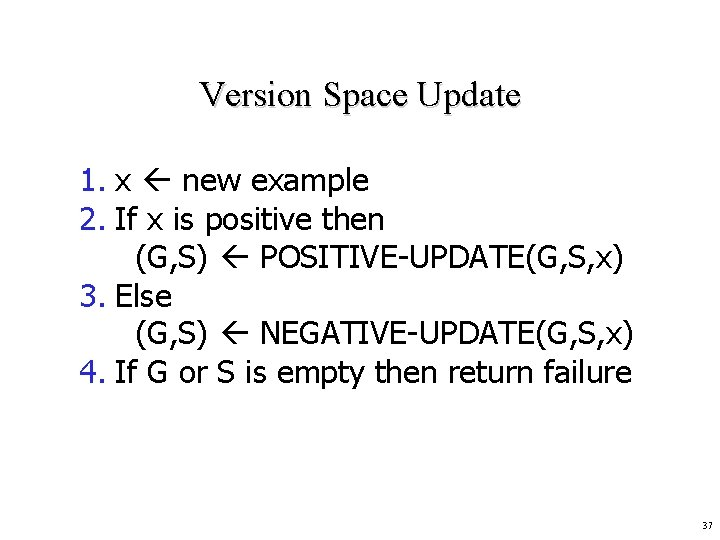Version Space Update 1. x new example 2. If x is positive then (G, S) POSITIVE-UPDATE(G, S, x) 3. Else (G, S) NEGATIVE-UPDATE(G, S, x) 4. If G or S is empty then return failure 37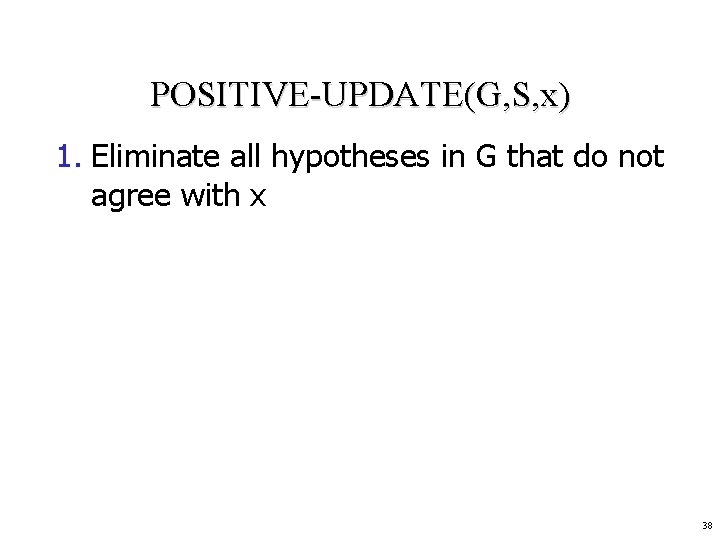POSITIVE-UPDATE(G, S, x) 1. Eliminate all hypotheses in G that do not agree with x 38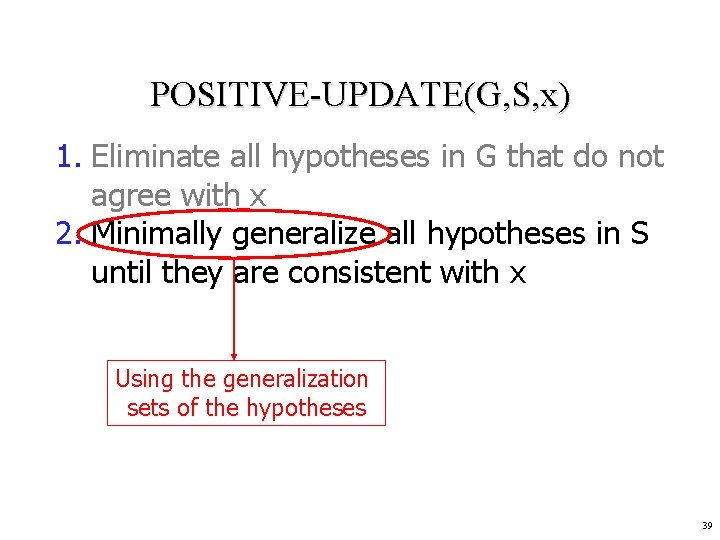POSITIVE-UPDATE(G, S, x) 1. Eliminate all hypotheses in G that do not agree with x 2. Minimally generalize all hypotheses in S until they are consistent with x Using the generalization sets of the hypotheses 39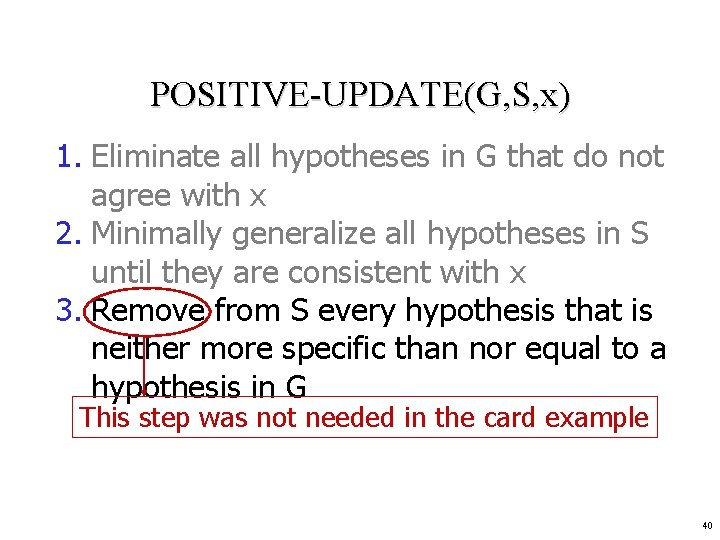POSITIVE-UPDATE(G, S, x) 1. Eliminate all hypotheses in G that do not agree with x 2. Minimally generalize all hypotheses in S until they are consistent with x 3. Remove from S every hypothesis that is neither more specific than nor equal to a hypothesis in G This step was not needed in the card example 40POSITIVE-UPDATE(G, S, x) 1. Eliminate all hypotheses in G that do not agree with x 2. Minimally generalize all hypotheses in S until they are consistent with x 3. Remove from S every hypothesis that is neither more specific than nor equal to a hypothesis in G 4. Remove from S every hypothesis that is more general than another hypothesis in S 5. Return (G, S) 41NEGATIVE-UPDATE(G, S, x) 1. Eliminate all hypotheses in S that do agree with x 2. Minimally specialize all hypotheses in G until they are consistent with (exclude) x 3. Remove from G every hypothesis that is neither more general than nor equal to a hypothesis in S 4. Remove from G every hypothesis that is more specific than another hypothesis in G 5. Return (G, S) 42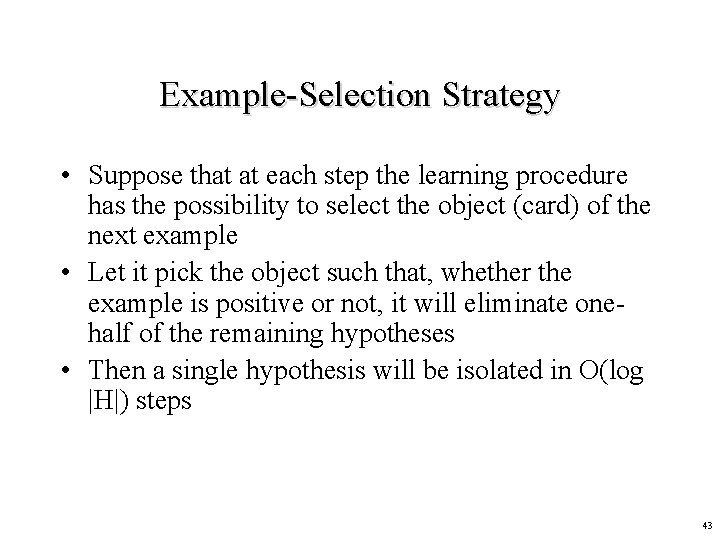Example-Selection Strategy • Suppose that at each step the learning procedure has the possibility to select the object (card) of the next example • Let it pick the object such that, whether the example is positive or not, it will eliminate onehalf of the remaining hypotheses • Then a single hypothesis will be isolated in O(log |H|) steps 43Example aa na • 9 ? • j ? ab a nb n 44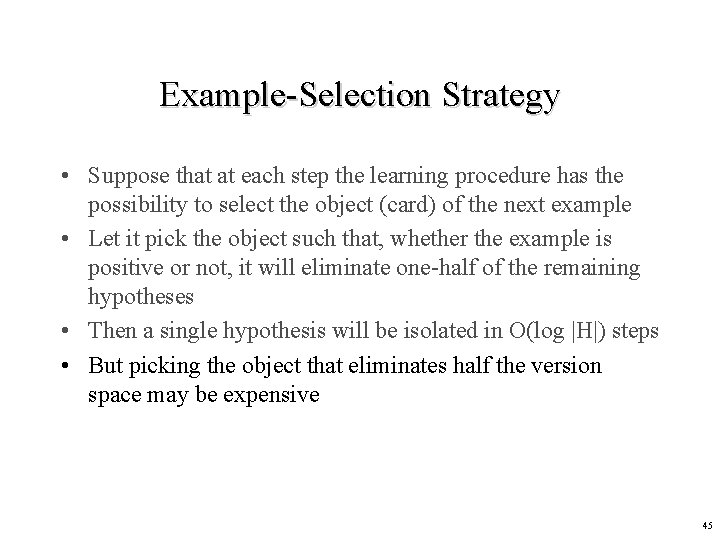Example-Selection Strategy • Suppose that at each step the learning procedure has the possibility to select the object (card) of the next example • Let it pick the object such that, whether the example is positive or not, it will eliminate one-half of the remaining hypotheses • Then a single hypothesis will be isolated in O(log |H|) steps • But picking the object that eliminates half the version space may be expensive 45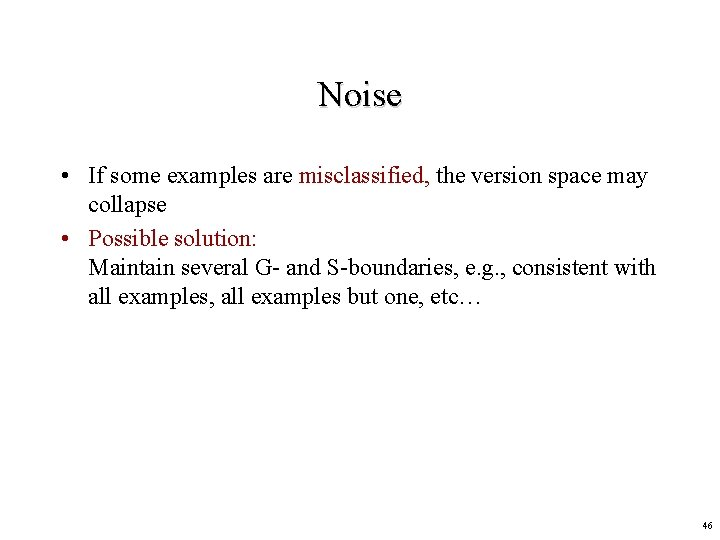Noise • If some examples are misclassified, the version space may collapse • Possible solution: Maintain several G- and S-boundaries, e. g. , consistent with all examples, all examples but one, etc… 46VSL vs DTL • Decision tree learning (DTL) is more efficient if all examples are given in advance; else, it may produce successive hypotheses, each poorly related to the previous one • Version space learning (VSL) is incremental • DTL can produce simplified hypotheses that do not agree with all examples • DTL has been more widely used in practice 47